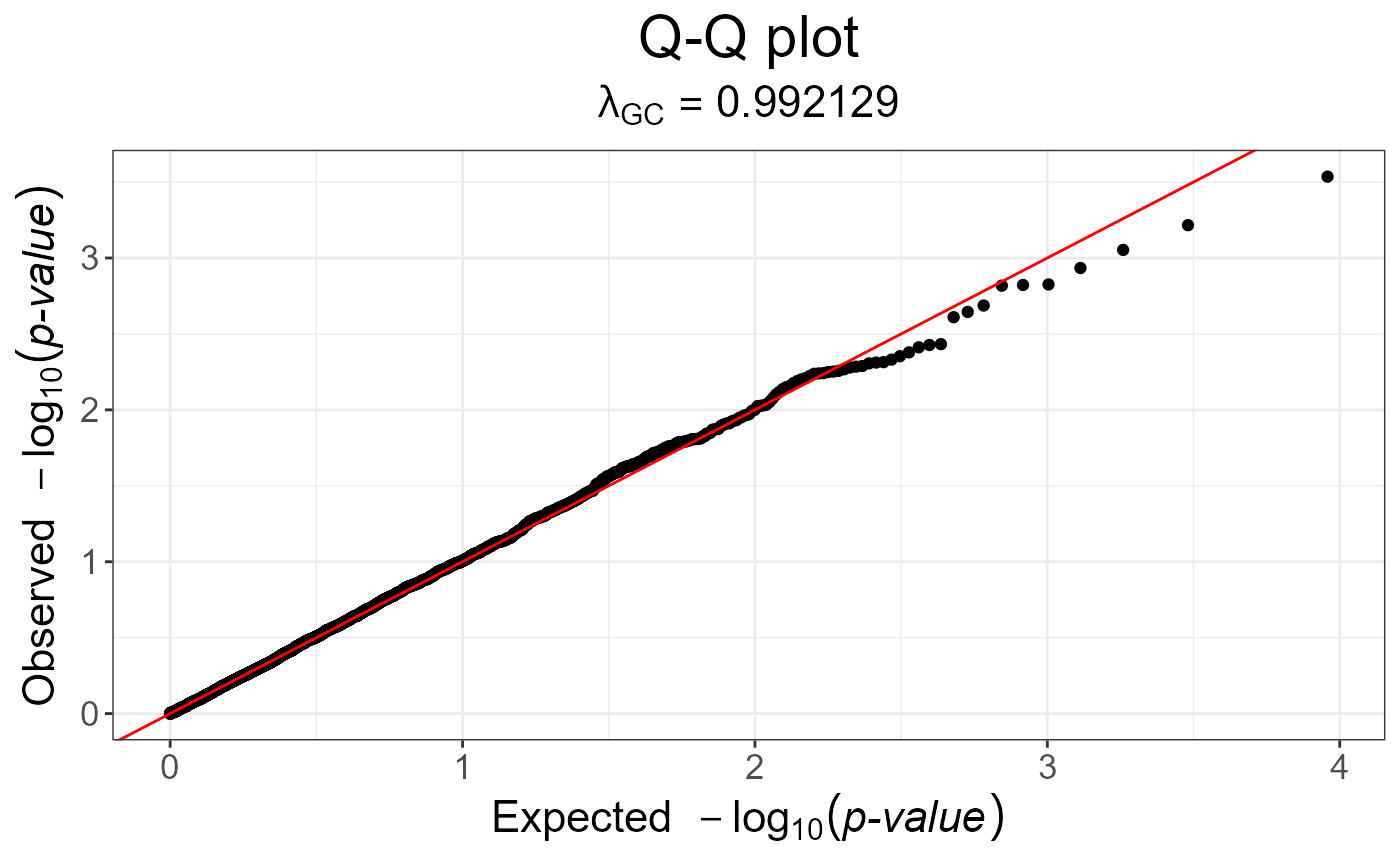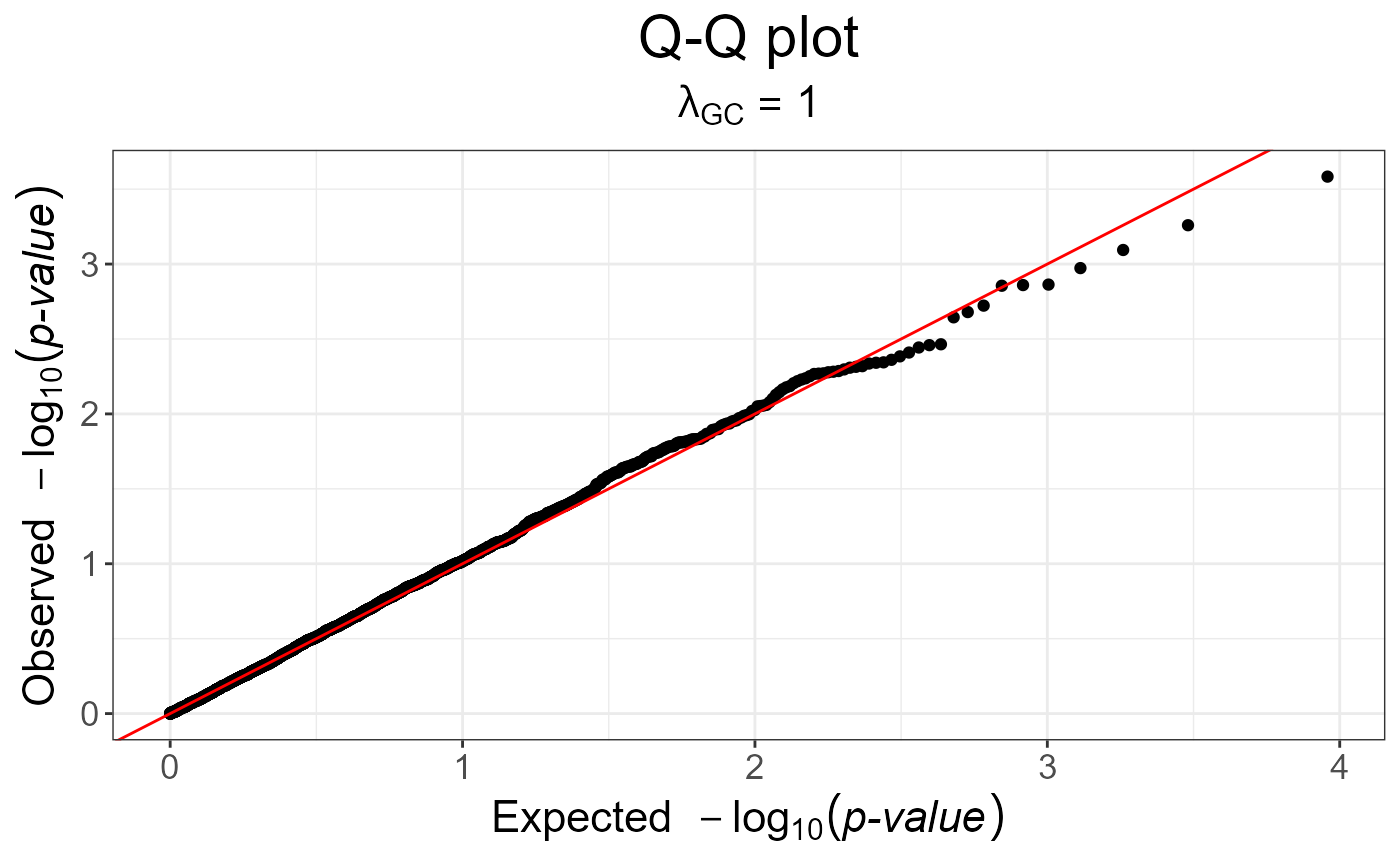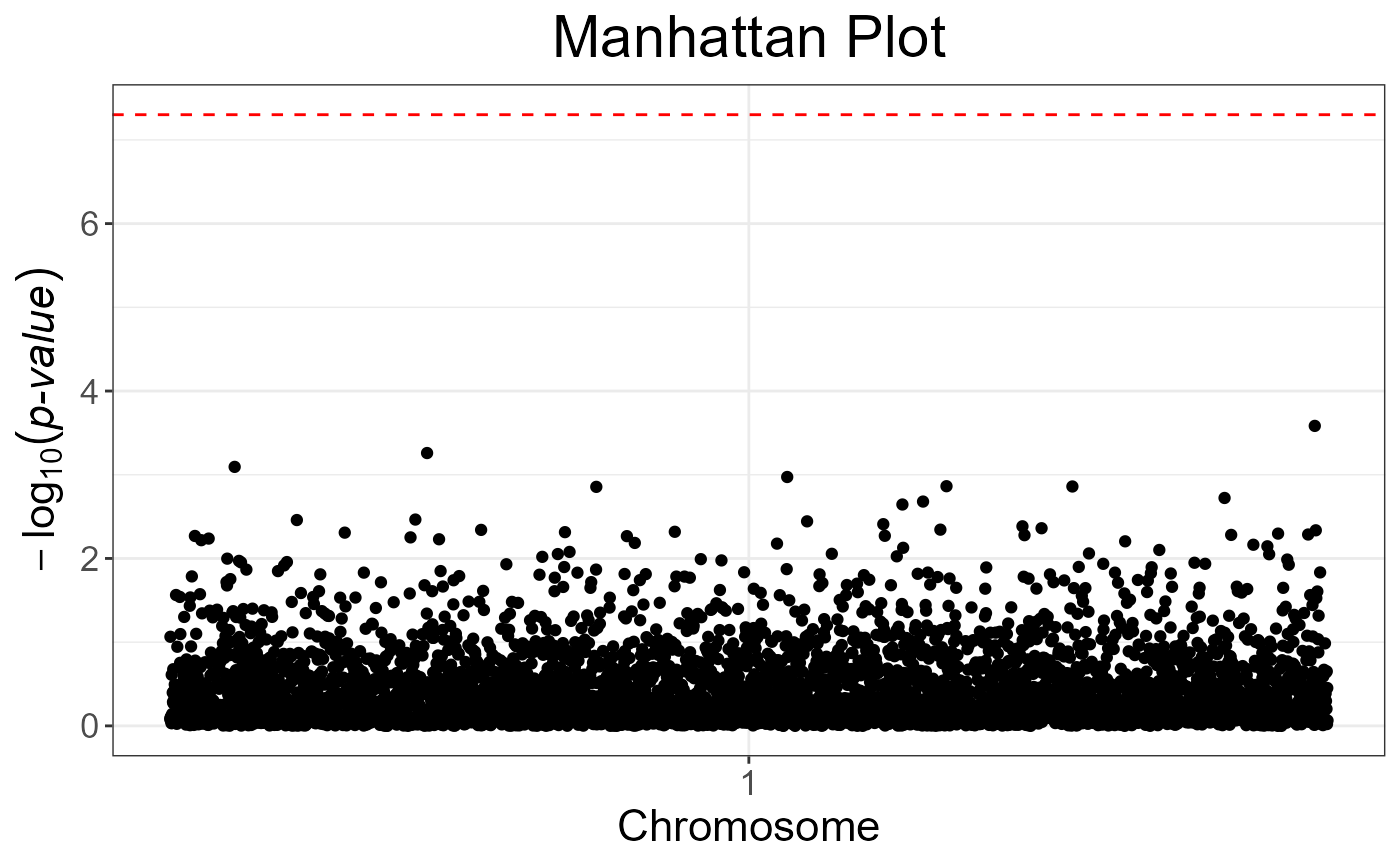Creates a quantile-quantile plot from p-values from a GWAS study.

snp_qq(gwas, lambdaGC = TRUE, coeff = 1)

## Arguments

gwas

A mhtest object with the p-values associated with each SNP. Typically, the output of big_univLinReg, big_univLogReg or snp_pcadapt.

lambdaGC

Add the Genomic Control coefficient as subtitle to the plot?

coeff

Relative size of text. Default is 1.

## Value

A ggplot2 object. You can plot it using the print method. You can modify it as you wish by adding layers. You might want to read this chapter

to get more familiar with the package ggplot2.

## Examples

set.seed(9)

test <- snp_attachExtdata()
G <- test$genotypes y <- rnorm(nrow(G)) gwas <- big_univLinReg(G, y) snp_qq(gwas)gwas_gc <- snp_gc(gwas) # change attr(gwas_gc, "transfo") snp_qq(gwas_gc)# The next plot should be prettier with a real dataset snp_manhattan(gwas_gc, infos.chr = test$map$chromosome, infos.pos = test$map$physical.pos)p <- snp_qq(gwas_gc) + ggplot2::aes(text = asPlotlyText(test$map))
if (FALSE) plotly::ggplotly(p, tooltip = "text")